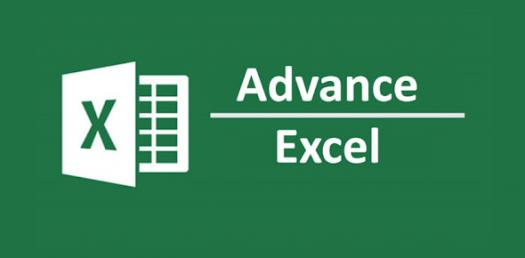# Excel Advance 1 Post Test

15 Questions | Total Attempts: 521SettingsSelect either True, False or I Don't Know

• 1.
Data can be imported from  (Data boleh diimport dari  )
• A.

• B.

• 2.
​Delimited is one of Lookup Formula (Delimited merupakan salah satu formula di dalam Lookup)
• A.

True

• B.

False

• 3.
Data layout can be change by using Paste Link option (Susun atur data boleh ditukar dengan cara Paste Link)
• A.

True

• B.

False

• 4.
Conditional Formatting is a formatting technique that applies a specified format to a cell or range based upon a set of creteria  (Conditional Formatting adalah teknik mengformat sel atau range berdasarkan set kriteria tertentu)
• A.

True

• B.

False

• 5.
By applying Range Name for list data could ease run any Function (Dengan mengaplikasikan Range Name bagi senarai data, memudahkan penggunaan pelbagai fungsi)
• A.

True

• B.

False

• 6.
What is Data Validation? (Apa itu Data Validation?)
• A.

Control data entered by user

• B.

Import data from system

• 7.
Protect Sheet and Protect Workbook option can be found in View Ribbon (Pilihan Protect Sheet dan Protect Workbook boleh didapati di ribbon View)
• A.

True

• B.

False

• 8.
By default cells in Microsoft Excel is unlocked. (Secara asasnya, sel di dalam Microsoft Excel tidak dikunci)
• A.

True

• B.

False

• 9.
Limitation of Column and Row for Excel 2007, 2010 and 2013?
• A.

XFD, 1,048,576

• B.

IV, 65,536

• 10.
VLOOKUP is one of function in Database Function (VLOOKUP merupakan salah satu fungsi di dalam kategori DATABASE)
• A.

True

• B.

False

• 11.
There are three types of LOOKUP functions, LOOKUP, VLOOKUP and CLOOKUP (Terdapat tiga jenis fungsi didalam LOOKUP, LOOKUP, VLOOKUP dan CLOOKUP)
• A.

True

• B.

False

• 12.
Examples of Conditional Formatting  (Contoh-contoh Conditional Formatting )
• A.

Data Bar, Colour Scale and Highlight Cell Rules

• B.

Colour Scale, Data Bar and Fill Colour

• C.

Font Colour, Cell Colour and Range Name Colour

• 13.
You can use Tracer Arrows or Watch Windows to create formula. (Anda boleh menggunakan Tracer Arrow atau Watch Windows untuk mencipta formula)
• A.

True

• B.

False

• 14.
You can create a chart as simple as press F12 key button (Anda boleh mencipta graf dengan semudah menekan kekunci F12)
• A.

True

• B.

False

• 15.
What is the answer for this formula?  (Apakah jawapan bagi formula berikut)=if(A1>=B1,"Good","Not Good")      ---> A1=90 , B1=90
• A.

Good

• B.

Not Good

Related TopicsBack to top